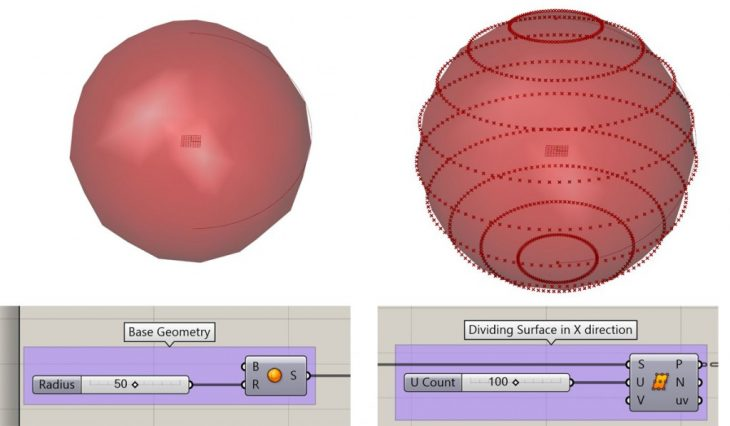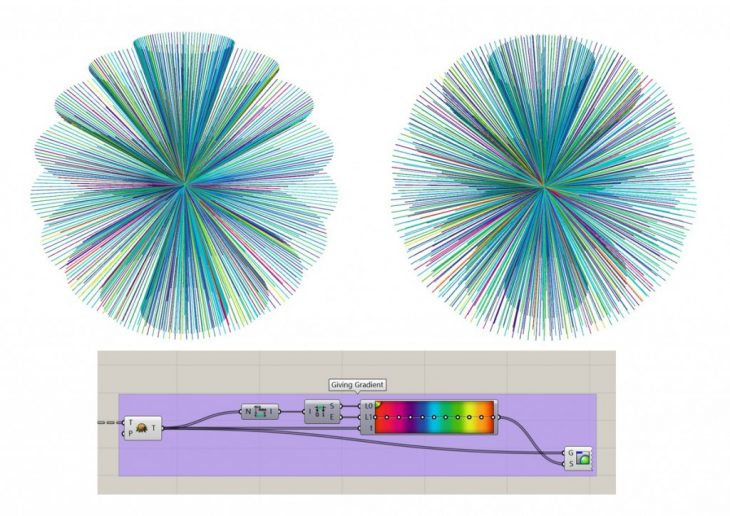COMPUTATIONAL DESIGN

GENERATIVE ALGORITHMS

CONGRUENCE

The objective was to apply computational design strategies as a form finding tool. The exercise was focused on a tridimensional manipulation of a simple sphere performed by combining multiple transformations. We were asked to do geometry manipulations : through euclidean transformation and through data list manipulation and also apply a gradient defining the local properties of the resulting single parts of the geometry with the overall.Sphere is of Radius 50 with the help of divide surface, the sphere is divided into several points only in X axis direction.Joining line from center to all the points that divide the surface. Then giving these lines some thickness via pipe variable function.Giving gradient to all the given set of pipes.

FINAL RESULTFINAL RENDER____________________________________________________________________________

TUTOR: ALDO SOLLAZZO & RODRIGO AGUIRRE

STUDENT: PRATYAKSH SHARMA

MaCT 2016-2017